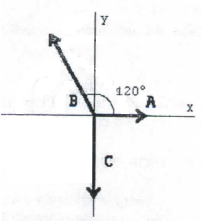# Problem: Find the magnitude and direction of the resultant R of the three vectors shown in the figure. The vectors have the following magnitudes; A = 7.9, B = 5.0, and C = 8.0. Express the direction of the vector sum by specifying the angle it makes with the positive x-axis with the counterclockwise angles taken to be positive.

🤓 Based on our data, we think this question is relevant for Professor Krasnoslobodtsev's class at UN OMAHA.

###### Problem Details

Find the magnitude and direction of the resultant R of the three vectors shown in the figure. The vectors have the following magnitudes; A = 7.9, B = 5.0, and C = 8.0. Express the direction of the vector sum by specifying the angle it makes with the positive x-axis with the counterclockwise angles taken to be positive.